﻿

### Derivations of abelian Lie algebra extensions

#### Abstract

Let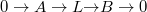$0 \rightarrow A\rightarrow L {\rightarrow} B \rightarrow 0$ be an abelian extension of Lie algebras. In this paper, we construct certain exact sequences which relate derivations with the Lie algebra cohomology group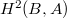$H^{2}(B,A)$, and apply them to study extending derivations of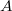$A$ and lifting derivations of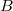$B$ to certain derivations of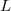$L$.

DOI Code: 10.1285/i15900932v39n2p71

Keywords: Derivation of Lie algebras; extensions of Lie algebras; second cohomology of Lie algebras; semidirect sum

Full Text: PDF